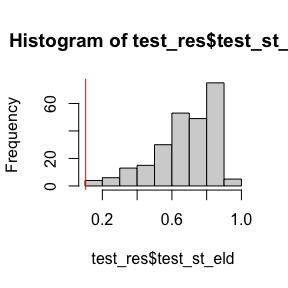# Running a Multivariate Point-Null Test

library(amp)

## Carrying out a test with a pre-built IC

Here, we will go through one of the examples from the paper for which we have already built an influence curve (IC). For a more formal introduction of influence curves, see this article. In this example, $$Y$$ is the outcome of interest, and $$X_1$$,…,$$X_d$$ are $$d$$ covariates measured on each (independent) individual. The parameter in this example is the pearson correlation coefficient between the outcome of interest and each covariate, that is $$\psi_i = \text{corr}(Y, X_1)$$. The null hypothesis we wish to test is that $$\psi_1 = \psi_2 =$$$$=\psi_d=0$$.

x_data <- matrix(rnorm(5000), ncol = 5)
y_data <- rnorm(1000) + 0.02 * x_data[, 2]
obs_data <- data.frame(y_data, x_data)

Here, we have already written a function that calculates both the parameter estimate and the IC for this parameter (we also choose to make no assumptions about the data generating mechanism).

amp::ic.pearson(obs_data, what = "est")
#> $est #> X1 X2 X3 X4 X5 #> [1,] -0.02897102 -0.02868844 -0.06009732 0.05895252 -0.007182061 cor(y_data, x_data) #> [,1] [,2] [,3] [,4] [,5] #> [1,] -0.02897102 -0.02868844 -0.06009732 0.05895252 -0.007182061 We are now ready to test the multivariate point null. test_res <- amp::mv_pn_test(obs_data, param_est = amp::ic.pearson, control = amp::test.control()) hist(test_res$test_st_eld)
abline(v = test_res$pvalue, col = "red")## Building an IC function yourself The derivation of influence functions for parameter estimators is quite technically challenging. However, once the IC and the parameter estimators have been derived, they can be used by this package. As an example, we will consider the influence function for the sample mean which is just $$x - \bar{x}$$. The function that is passed to the mv_pn_test will always take three arguments. • The first argument (observ) should be the observed data • The second argument (what) will specify if the function should return the estimate (“est”), the influence curve (“ic”), or both (“both”). • The third argument control takes any other arguments used to control the function. Even if your function does not use other arguments, the function definition should still contain control = NULL. The object returned by the function should be a list which contains • "est" if what is either "est" or "both" • "ic" if what is either "ic" or "both" ic.mean <- function(observ, what = "both", control = NULL){ if (!(what %in% c("ic", "est", "both"))) { stop("what must be one of ic (influence curve), est (estimate), or both") } ret <- list() if (what %in% c("ic", "both")) { col_means <- colMeans(x = observ) infl <- sweep(x = observ, MARGIN = 2, STATS = col_means, FUN = "-") ret$ic <- infl
}
if (what %in% c("est", "both")) {
ret$est <- colMeans(x = observ) } return(ret) } Now that we have our newly defined IC, we can pass it to the testing function. We first create a new dataset for which we are interested in testing if each covariate has mean zero: set.seed(100) obs_data_mean1 <- matrix(rnorm(n = 500) + rep(c(0, 0, 0, 0, 0.01), each = 100), ncol = 5, byrow = FALSE) res_1 <- amp::mv_pn_test(obs_data_mean1, param_est = ic.mean, control = amp::test.control()) obs_data_mean2 <- matrix(rnorm(n = 500) + rep(c(0, 0, 0.32, 0, 0.07), each = 100), ncol = 5, byrow = FALSE) res_2 <- amp::mv_pn_test(obs_data_mean2, param_est = ic.mean, control = amp::test.control()) print(c(res_1$pvalue, res_2$pvalue)) #>  0.788 0.080 In cases where control will modify the behavior of the IC or parameter estimation, these arguments should be appended to the list of already created arguments in amp::test.control() and passed to the control argument of the mv_pn_test. ### A very minor note When passing control arguments to the param_est function, it is recommended that if needed, these functions explicitly pull these arguments out of control before passing them to a nested function: ## Correct usage nested_fun1 <- function(x) return(x) ic.mean1 <- function(observ, what = "both", control = NULL){ if (!(what %in% c("ic", "est", "both"))) { stop("what must be one of ic (influence curve), est (estimate), or both") } ret <- list() if (what %in% c("ic", "both")) { mult <- nested_fun1(control$extra_arg)
col_means <- mult * colMeans(x = observ)
infl <- sweep(x = observ, MARGIN = 2,
STATS = col_means, FUN = "-")
ret$ic <- infl } if (what %in% c("est", "both")) { ret$est <- colMeans(x = observ)
}
return(ret)
}

test1 <- amp::mv_pn_test(obs_data_mean1, param_est = ic.mean1,
control = c(amp::test.control(),
"extra_arg" = 2))

## Incorrect usage
nested_fun2 <- function(x) return(x$extra_arg) ic.mean2 <- function(observ, what = "both", control = NULL){ if (!(what %in% c("ic", "est", "both"))) { stop("what must be one of ic (influence curve), est (estimate), or both") } ret <- list() if (what %in% c("ic", "both")) { mult <- nested_fun2(control) col_means <- mult * colMeans(x = observ) infl <- sweep(x = observ, MARGIN = 2, STATS = col_means, FUN = "-") ret$ic <- infl
}
if (what %in% c("est", "both")) {
ret\$est <- colMeans(x = observ)
}
return(ret)
}
test2 <- amp::mv_pn_test(obs_data_mean1, param_est = ic.mean2,
control = c(amp::test.control(),
"extra_arg" = 2))
#> Warning in .checkargs(obs_data, param_est, control): Some arguments appear not to be used by the test.  These are:
#>  extra_arg
#>  To avoid this warning, explicitly call these arguments in your param_est function (rather than just passing all control arguments to another function.